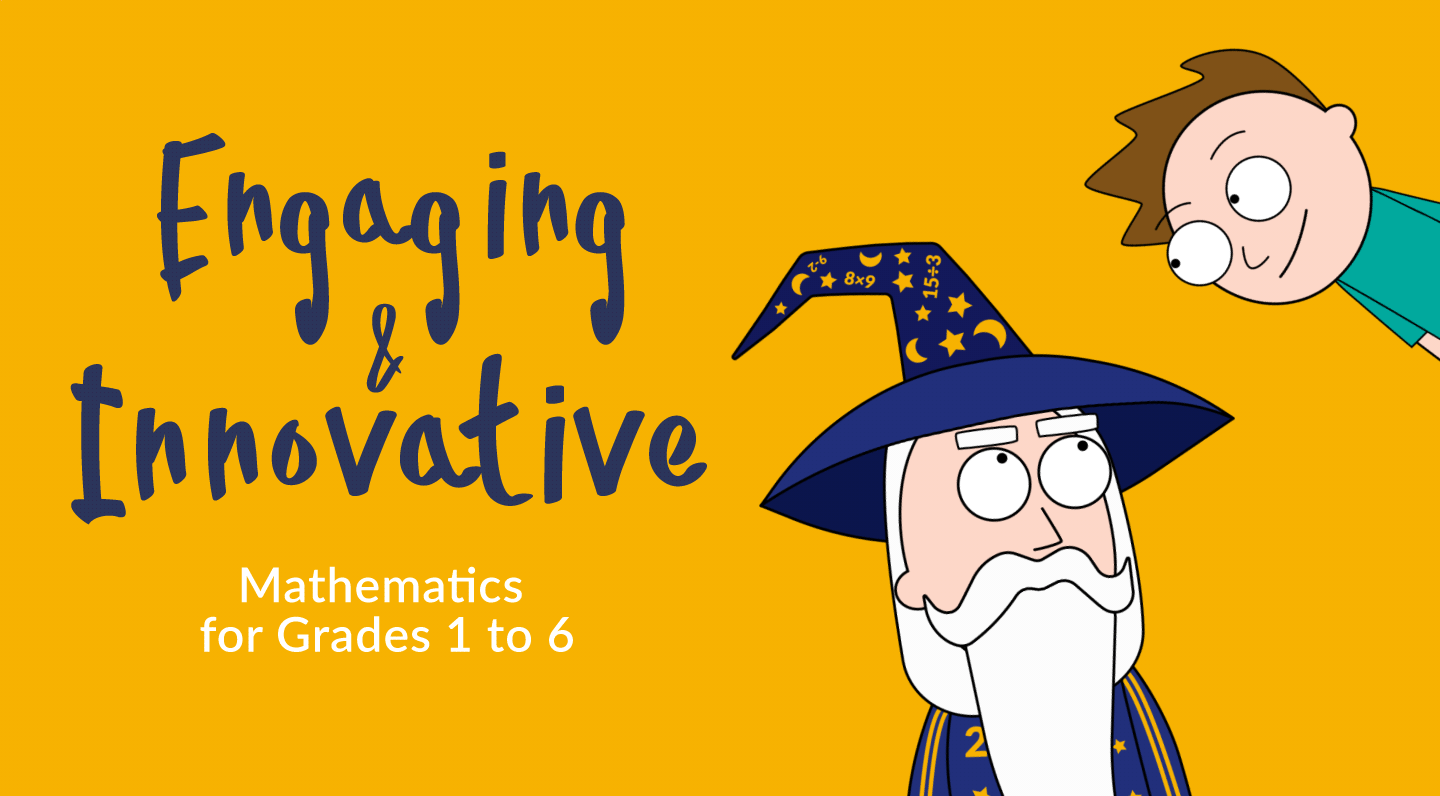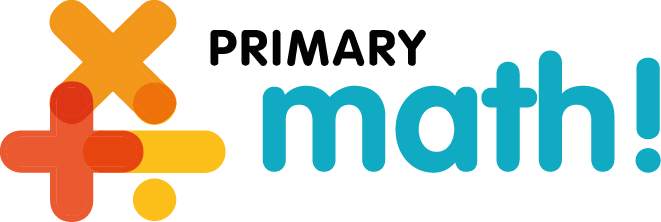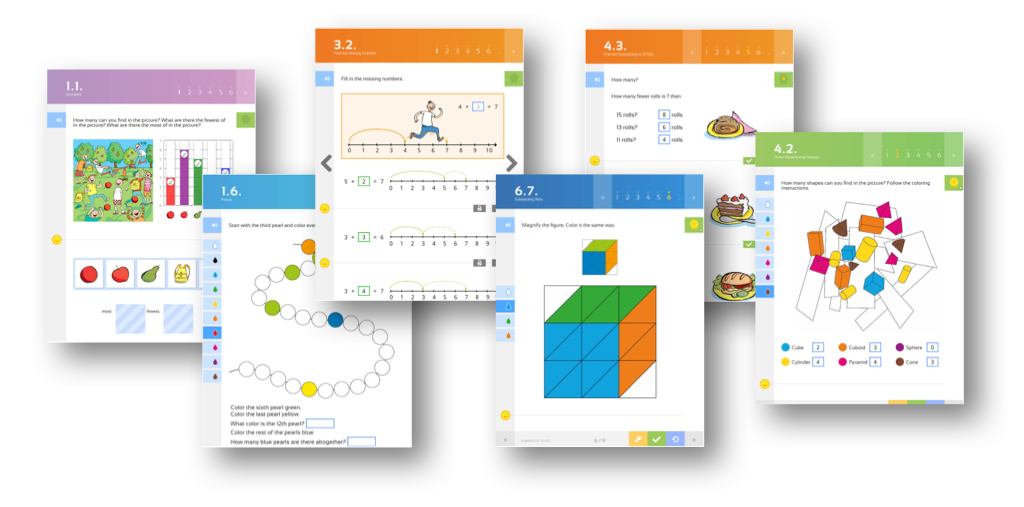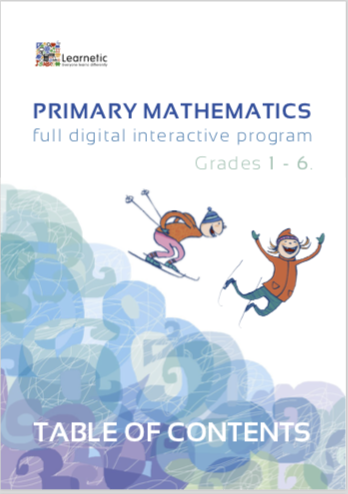# The most innovativeINTERACTIVE MATH CURRICULUMfor Grades 1 to 6

.

PrimaryMath! is a comprehensive set of highly interactive, digital lessons for children’s numeracy skills at the initial levels of their math education. It covers 6 years of regular math learning and helps develop pupil’s creativity and their math perception in general.

PrimaryMath! offers a proven, innovative teaching methodology, recognized worldwide and based on:

• experimental math,
• multiple methods,
• visualization of mathematical thinking,
• variety of strategies,
• individual learning process,
• language of mathematics.

.

.### Engaging Activities

Over 2,600 engaging math activities in 320 topics for grades 1 – 3; over 3,600 activities in 344 topics for grades 4 – 6.

### Methodology

Based on Finnish publisher’s textbooks and supplementary materials referring to the renowned Finnish methodology.

### Home & School

An excellent solution for individual work at home and class activities – at school or remotely.

### Teacher’s Materials

Includes additional instructional materials for teachers helping them to practically apply the innovative teaching approach.

### Reporting

Full reporting system for teachers and parents, including the number of mistakes, the number of checks, and the time spent on each exercise and each lesson.

### Common Core

High level of matching of the Primary Math! package with the Common Core Standards for Mathematics.

### Feedback

Engaging and stimulating feedback system tailored to pupils’ age.

### On-line / Off-line

Accessible from any mobile or stationary device on-line or off-line.

No. of Lessons
.

No. of ePages
.

416
464
416
424
504
504
608
650
548
635
650
559

#### 1. Count and Compare Numbers

1.0. Quantities
1.1. Numbers
1.2. Numbers and Numerals
1.3. The Number Line
1.4. How Many Altogether?
1.5. More Than, Fewer Than
1.6. Equal Number
1.7. Focus
1.8. What I Have Learned
1.9. Draw

#### 2. Numbers 0–5

2.0. Numbers 0–5
2.1. Numbers 0 and 1
2.2. Numbers 2 and 3
2.3. Numbers 4 and 5
2.4. Comparing Numbers
2.5. Equal to
2.7. Addition on a Number Line
2.8. Subtraction
2.9. Subtracting on a Number Line
2.10. Practice Subtraction
2.12. Commutative Property
2.13. Focus
2.14. What I Have Learned
2.15. Draw

#### 3. Numbers 6–10

3.0. Numbers 6–10
3.1. Numbers 6 and 7
3.2. Find the Missing Number
3.3. Counting Money
3.5. Numbers 8 and 9
3.6. Comparing Numbers
3.8. Number 10
3.10. Two-Step Subtraction
3.11. Focus
3.12. What I Have Learned
3.13. Draw

#### 4. Geometry and Measurement

4.0. Geometry and Measurement
4.1. Shapes and Objects in Three dimensions
4.2. Three-Dimensional Shapes
4.3. Two-Dimensional Shapes
4.4. Draw Two-Dimensional Shapes
4.5. Build and Draw
4.6. Double and Half
4.7. Measure Length
4.8. Points and Line Segments
4.9. Focus
4.10. What I Have Learned
4.11. Draw

#### 1. Numbers up to 20

1.0. Numbers up to 20
1.1. Numbers
1.2. Numbers and Numerals
1.3. Comparing Numbers
1.4. Ordinal Numbers
1.5. Odd and Even Numbers
1.6. Focus
1.7. What I Have Learned
1.8. Draw

#### 2. Telling Time

2.0. Telling Time
2.1. The Clock Face
2.2. Time to the Hour
2.3. Time to the Half Hour
2.4. Earlier or Later
2.5. Focus
2.6. What I Have Learned
2.7. Draw

3.3. Add to a Two-Digit Number
3.4. Add to 10 First (from 8 or 9)
3.5. Add to 10 First (from 6 or 7)
3.6. Commutative Property
3.7. Focus
3.8. What I Have Learned
3.9. Draw

#### 4. Subtraction within 20

4.0. Subtraction within 20
4.1. Subtracting from a Two-Digit Number
4.2. Subtract to 10 First
4.3. Practice Subtracting to 10 first
4.5. Word Problems
4.6. Focus
4.7. What I Have Learned
4.8. Draw

#### 5. Mathematics and Money

5.0. Mathematics and Money
5.1. Coins and Notes
5.2. Estimate the Price
5.3. How much altogether?
5.4. How Much Is Left?
5.5. Compare Prices
5.6. Storytelling
5.7. Focus
5.8. What I Have Learned
5.9. Draw

#### 6. Numbers up to 100

6.0. Numbers up to 100
6.1. Place Value
6.2. Number Sequences
6.3. Compare Numbers
6.4. Apply What You Know
6.7. Subtracting Tens
6.8. Subtraction within 99
6.9. Focus
6.10. What I Have Learned
6.11. Draw

#### 1. Numbers up to 20

1.0. Numbers up to 20
1.1. Ones and Tens
1.2. Comparing Numbers on a Number Line
1.5. Subtraction
1.6. Practice Subtraction
1.8. Focus
1.9. What I Have Learned
1.10. Draw

#### 2. Numbers up to 100

2.0. Numbers up to 100
2.1. Number Sequences
2.2. Odd and Even Numbers
2.5. Subtracting Two-Digit Numbers
2.6. Practice Subtraction
2.7. Compare Numbers
2.8. Word Problems
2.9. Counting Coins
2.10. Focus
2.11. What I Have Learned
2.12. Draw

#### 3. Multiplication

3.0. Multiplication
3.2. Picture Multiplication
3.3. Word Problems
3.4. Multiplying by 2
3.5. Practice Multiplying by 2
3.6. Multiplying by 4
3.7. Practice Multiplying by 4
3.8. Multiplying by 5
3.9. Practice Multiplying by 5
3.10. Multiplying by 10
3.11. Multiplying by 3
3.12. Practice Multiplying by 3
3.13. Practice Multiplications
3.14. Focus
3.15. What I Have Learned
3.16. Draw

#### 4. Geometry and Measurement

4.0. Geometry and Measurement
4.1. Three-Dimensional Shapes
4.2. Perspective
4.3. Two-Dimensional Shapes
4.4. Geometric Terms
4.5. Measure Length
4.6. Perimeter
4.7. Area
4.8. Symmetry
4.9. Focus
4.10. What I Have Learned

#### 1. Measuring Tools

1.0. Measurement
1.1. Time to the Hour and the Half Hour
1.2. 15 Minutes
1.3. Elapsed Time
1.4. To and Past
1.5. Length
1.6. Millimetres and Centimetres
1.7. Metres
1.8. Kilometres
1.9. Mass
1.10. Grams and Kilograms
1.11. Volume
1.12. Liters and Deciliters
1.13. Focus
1.14. What I Have Learned
1.15. Draw

#### 2. Multiplication and Division

2.0. Multiplication and Division
2.1. Revise Multiplying by 2 and 4
2.2. Revise Multiplying by 5 and 10
2.3. Revise Multiplying by 3
2.4. Revise Multiplying by 3
2.5. Partitive Division
2.6. Quotative Division
2.7. Half, a Quarter and a Third
2.8. Divide into Equal Parts
2.9. Remember?
2.10. Focus
2.11. What I Have Learned
2.12. Draw

#### 3. Numbers up to 1 000

3.0. Numbers up to 1 000
3.1. Hundreds
3.2. Hundreds and Tens
3.3. Hundreds, Tens and Ones
3.4. Number Sequences
3.5. The Number Line
3.6. Comparing Numbers
3.10. Subtracting Hundreds
3.11. Subtracting Tens
3.12. Subtracting Ones
3.13. The Standard Algorithm of Addition
3.14. The Standard Algorithm of Subtraction
3.15. Euros and Cents
3.16. Focus
3.17. What I have Learned
3.18. Draw

#### 4. Investigate and Experiment

4.0. Investigate and Experiment
4.1. Dividing into Equal Parts
4.2. Folding Paper
4.3. Throwing Dice
4.4. Math Problems

#### 1. Addition and Subtraction with Numbers up to 100

1.0. Addition and Subtraction with Numbers up to 100
1.1. Place Value
1.2. Tens
1.3. Composing Tens
1.4 Subtraction within 100
1.5. Decomposing Tens
1.6. Sum and Difference
1.8. Focus
1.9. What I Have Learned

#### 2. Multiplication

2.0. Multiplication
2.1. Revise Multiplication Tables
2.2. Commutative Property
2.3. Multiplying by 6
2.4. Multiplying by 7
2.5. Multiplying by 8
2.6. Multiplying by 9
2.7. Order of Operations
2.9. Focus
2.10. What I Have Learned

#### 3. Division

3.0. Division
3.1. Partitive Division
3.2. Dividing by 2 – 5
3.3. Dividing by 6 – 10
3.4. Relating Multiplication and Division
3.5. Quotative Division
3.6. The Fraction Bar
3.7. Division with Remainders
3.8. Practice Division with Remainders
3.10. Focus
3.11. What I Have Learned

#### 4. Numbers up to 1 000

4.0. Numbers up to 1 000
4.1. Hundreds, Tens and Ones
4.2. Number Sequences
4.3. Comparing Numbers on a Number Line
4.4. Partitioning Numbers up to 1 000
4.6. Adding Numbers with Three Digits
4.7. Composing Hundreds
4.8. Subtraction within 1 000
4.9. Adding Numbers with Three Digits
4.10. Decomposing Hundreds
4.11. The Standard Algorithm of Addition
4.13. The Standard Algorithm of Subtraction
4.14. Subtraction with Regrouping
4.15. Subtraction Across Zeros
4.17. Focus
4.18. What I Have Learned

#### 5. Geometry

5.0. Geometry
5.1. Three-dimensional Shapes
5.2. Angles
5.3. Triangles
5.5. Symmetry
5.6. Perimeter
5.7. Area
5.9. Focus
5.10. What I Have Learned

#### 1. Measurement

1.0. Measurement
1.1. 24 Hours
1.2. Minutes
1.3. Elapsed Time
1.4. Units of Length
1.6. Kilometres and Metres
1.7. Mass
1.8. Kilograms and Grams
1.9. Volume
1.10. Liters and Deciliters
1.12 Focus
1.13. What I Have Learned

#### 2. Fractions and Decimals

2.0. Fractions and Decimals
2.1. Dividing into Equal Parts
2.2. Fractions
2.3. Writing a Fraction
2.4. Fractions on a Number Line
2.6. Subtracting Fractions
2.7. Equal Parts of a Whole
2.8. A Fraction of a Group
2.9. From Fractions to Decimals
2.10. Decimal Numbers
2.11. Applying Decimal Numbers
2.12. Decimal Numbers and Money
2.14. Focus
2.15. What I Have Learned

#### 3. Multiplication with large numbers

3.0. Multiplication with Large Numbers
3.1. Multiply by 10 and 100
3.2. Multiply by Tens and Hundreds
3.3. Multiplying a Two-Digit Number
3.4. Multiplying Large Numbers
3.5. Long Multiplication
3.6. Multiplication with Regrouping
3.7. Practice Regrouping
3.9. Focus
3.10. What I Have Learned

#### 4. Numbers up to 10 000

4.0. Numbers up to 10 000
4.1. Ten Thousand
4.2. Thousands
4.3. Thousands and Hundreds
4.4. Thousands, Hundreds and Tens
4.5. Thousands, Hundreds, Tens and Ones
4.6. Compare Numbers
4.8. Focus
4.9. What I Have Learned

#### 5. Addition and Subtraction within 10 000

5.0. Addition and Subtraction within 10 000
5.1. Partitioning Numbers up to 10 000
5.4. Subtracting Hundreds
5.5. Subtraction with Numbers up to 10 000
5.6. The Standard Algorithm of Addition
5.7. The Standard Algorithm of Subtraction
5.8. Subtraction Across Zeros
5.10. Focus
5.11. What I Have Learned

#### 1. Addition and Subtraction within 10 000

1.1. Numbers up to 10 000
1.2. Partitioning Numbers up to 10 000
1.3. Approximation
1.4. Rounding
1.7. Revise Subtraction
1.8. Decompose Numbers for Subtraction
1.9. Focus
1.10.What I’ve Learned?
1.11. Draw

#### 2. Ten Thousands, Hundred Thousands and Millions

2.1. Ten Thousands, Hundred Thousands and Millions
2.2. Ten Thousands
2.3. Compare Numbers
2.4. Rounding
2.5. Subtracting Ten, Hundred, Thousand and Ten thousand
2.6. The Standard Algorithm of Addition
2.7. The Standard Algorithm of Subtraction
2.8. Subtraction Across Zeros
2.9. Hundred Thousands
2.10. Millions
2.11. Examining Millions
2.13. Focus
2.14. What I’ve Learned?
2.15. Draw

#### 3. Multiplication and Division

3.1. Multiplication and Division
3.2. Revising Multiplication
3.3. Order of Operations and Parentheses
3.4. Relating Multiplication and Division
3.5. Quotative Division
3.6. Division with Remainders
3.7. Order of Operations and Parentheses 2
3.8. mSolve Math Problems with a Calculator
3.9. Focus
3.10. What I’ve Learned?
3.11. Draw

#### 4. Multiplying Large Numbers

4.1. Multiplying Large Numbers
4.2. Multiplying by 10, 100 and 1 000
4.3. Multiplying Numbers Ending with Zero
4.4. Multiplying Tens and Hundreds
4.5. Multiplying a Two-Digit Number
4.6. Multiplying Three Factors
4.7. Multiplying Large Numbers
4.8. Long Multiplication
4.9. Multiplication with Regrouping
4.10. Focus
4.11. What I’ve Learned
4.12. Draw

#### 5. Geometry

5.1. Geometry
5.2. Three-dimensional Shapes
5.3. From Three Dimensions to Two Dimensions
5.4. Point, Line and Line Segment
5.5. Angles
5.6. Polygons
5.7. Tangram
5.8. Perimeter
5.9. Area
5.10. Coordinate Plane
5.11. Focus
5.12. What I’ve Learned
5.13. Draw

#### 1. Measurement

1.1. Years and Ages
1.2. Days and Months
1.3. Millimetres, Centimetres and Metres
1.4. Decimetres
1.5. Metres and Kilometres
1.6. Grams and Kilograms
1.7. Kilograms and Tonnes
1.8. Decilitres and Litres
1.9. Millilitres and Centilitres
1.10. Apply What You Know
1.11. Focus
1.12. What I’ve Learned
1.13. Draw

#### 2. Dividing Large Numbers

2.1. Relating Division and Multiplication
2.2. Division with Remainders
2.3. Short Division
2.4. Short Division with Remainders
2.5. Short Division with Carrying
2.6. Long Division
2.7. Practice Long Division
2.8. Zeros in the Quotient
2.9. Long Division with Remainders
2.10. Division Word Problems
2.11. Focus
2.12. What I’ve Learned
2.13. Draw

#### 3. Fractions

3.1. Fractions
3.2. Fractions as Division
3.3. From Fractions to Mixed Numbers
3.4. Compare Fractions
3.7. Subtracting Mixed Numbers
3.8. Subtracting Mixed Numbers with Regrouping
3.9. Apply What You Know
3.10. Focus
3.11. What I’ve Learned
3.12. Draw

#### 4. Decimals

4.1. From Fractions to Decimals
4.2. Tenths
4.3. Number Sequences: Tenths
4.4. Hundredths
4.5. Number Sequences: Hundredths
4.6. Rounding Decimals
4.7. Paying in Cash
4.10. Subtracting Tenths
4.11. Subtracting Hundredths
4.12. Apply What You Know
4.13. Focus
4.14. What I Have Learned
4.15. Draw

#### 5. Real-life Math

5.1. Time Usage
5.2. Waste Disposal
5.3. Forest Trees
5.4. The Cost of a Burger
5.5. Regular Patterns
5.6. Building a Birdhouse

#### 1. Place Value

1.1. Place Value
1.2. Revise Place Value
1.3. Multiply and Divide Numbers that End with Zero
1.4. Comparing Tens of Thousands on a Number Line
1.5. Partitioning Numbers up to 100 000
1.6. Tens of Millions
1.7. Large Numbers on a Number Line
1.8. Partitioning Numbers between 100 000 and 10 000 000
1.9. Apply What You Know
1.10. Focus
1.11. What I Have Learned

#### 2. Large Numbers and Negative Numbers

2.1. Large Numbers and Negative Numbers
2.4. Subtracting Whole Numbers
2.5. Addition and Subtraction on a Number Line
2.6. Count Millions
2.7. Standard Algorithms of Addition and Subtraction
2.8. Applying Addition and Subtraction Skills
2.9. The Celsius Scale of Temperature
2.10. Negative Numbers on a Number Line
2.11. Apply Negative Numbers
2.12. Focus
2.13. What I’ve Learned?

#### 3. Decimal Place Value

3.1. Revise Decimal Place Value
3.2. Decimal Place Value
3.3. Decimals on a Number Line
3.4. Rounding Decimals
3.5. Money
3.7. Adding Decimals Using the Standard Algorithm
3.9. Subtracting Decimals
3.10. Subtracting Decimals Using the Standard Algorithm
3.11. Practice Subtracting Decimals
3.12. Multiplying Decimals by 10, 100 and 1 000
3.13. Dividing Decimals by 10, 100 and 1 000
3.14. Multiplying Decimals
3.15. Dividing Decimals
3.16. Focus
3.17. What I’ve Learned?

#### 4. Geometry

4.1. Geometry
4.2. Three-Dimensional Shapes
4.3. Properties of Three-Dimensional Shapes
4.4. Angles
4.6. Area and Perimeter
4.7. The Area of a Parallelogram
4.8. The Area of a Triangle
4.9. Ratio and Similarity
4.10. Scale
4.11. Reflection and Rotation
4.12. Circle
4.13. Focus
4.14. What I’ve Learned?

#### 1. Multiplication and Division

1.1. Multiplying and Dividing
1.2. Multiplying Two-digit Numbers
1.3. Multiplication and Place Value
1.4. Order of Operations
1.5. Long Multiplication
1.6. Multipliers with Two Digits
1.7. Short Division
1.8. Adding Zeros when Dividing Decimals
1.9. Long Division
1.10. Applying Multiplication and Division Skills
1.11. Applying the Order of Operations
1.12. Focus
1.13. What I’ve Learned

#### 2. Fractions and Percentages

2.1. Fractions and Mixed Numbers
2.2. Divisibility
2.3. Simplifying Fractions
2.4. Adding and Subtracting Fractions with the Same Denominator
2.5. Least Common Denominator
2.6. Adding and Subtracting Fractions with Different Denominators
2.7. Multiplying Fractions by Integers
2.8. Finding a Fraction of an Amount
2.9. Dividing Fractions by Whole Numbers
2.10. Applying Fractions
2.11. Converting Between Decimals and Fractions
2.12. Percentages
2.13. Focus
2.14. What I’ve Learned

#### 3. Time and Measurement

3.1. Calculating Time
3.2. Units of Time
3.3. Elapsed Time
3.4. Units of Length
3.6. Units of Mass
3.7. Units of Volume
3.8. Applying Units of Measurement
3.9. Area
3.10. Find an Area
3.11. Focus
3.12. What I’ve Learned

#### 4. Statistics

4.1. Interpreting Timetables
4.2. Interpreting Tables
4.3. Coordinate Plane
4.4. Practice on the Coordinate Plane
4.5. Bar Charts and Line Graphs
4.6. Use Bar Charts and Line Graphs
4.7. Pie Charts
4.8. Probability
4.9. Mean
4.10. Median and Mode
4.11. Focus
4.12. What I’ve Learned

#### 1. Basic Operations

1.1. Basic Operations
1.2. Place Value
1.3. Rounding
1.4. Solving Word Problems
1.5. Adding and Subtracting Decimal Numbers
1.6. Divisibility
1.7. Relating Multiplication and Division
1.8. Dividing and Multiplying Decimals by a Whole Number
1.9. Dividing Decimals and Adding Zeros
1.10. Multiplying Decimals
1.11. Solve Word Problems: Multiplying Decimals
1.12. Unit Price
1.13. Focus
1.14. What I’ve Learned?

#### 2. Fractions, Decimals and Percentages

2.1. Fractions, Decimals and Percentages
2.2. Fraction, Decimal and Percentage
2.3. Percentage
2.4. Find simple percentages
2.5. Apply percentages
2.6. Common denominator
2.8. Adding and Subtracting Mixed Numbers
2.9. Multiplying Fractions
2.10. Dividing Fractions by Whole Numbers
2.11. Finding a Percentage
2.12. Revise and Apply
2.13. Focus
2.14. What I’ve Learned?

#### 3. Integers and Mental Math Strategies

3.1. Integers and Mental Math Strategies
3.2. Integers on a Number Line
3.3. Applying Integers
3.4. Everyday Negative Integers
3.5. Multiply and Divide by 1 000, 100, 10 and 0,1
3.6. Multiplication and Division Strategies
3.7. The Distributive Law
3.8. Quotative Division
3.9. Multiply and Divide by 5, 50 and 500
3.10. Converting Currency
3.11. Solving Word Problems
3.12. Solving Word Problems 2
3.13. Focus
3.14. What I’ve Learned?

#### 4. Statistics

4.1. Statistics
4.2. Four Quadrants of the Coordinate Plane
4.3. Write and Graph Coordinates
4.4. Write Probabilities as Fractions and Percentages
4.5. Pie Charts
4.6. Mean
4.7. Median, Mode and Range
4.8. Bar Graphs
4.9. Work with Bar Graphs
4.10. Histogram
4.11. Line Graphs 12. Apply Statistics
4.12. Focus
4.13. What I’ve Learned?

#### 1. Algebraic Thinking

1.1. Inverse Operations
1.2. Multiplication and Division Strategies 1
1.3. Multiplication and Division Strategies 2
1.4. Equations
1.5. Solve Equations
1.6. Word Problems and Writing Equations
1.7. Logical Reasoning
1.8. Number Lines and Codes
1.10. Dependent Variables
1.12. Area and Exponents
1.13. Exponent
1.14. Apply
1.15. Focus
1.16. What I’ve Learned

#### 2. Geometry and Measurement

2.1. Circle
2.2. Symmetry and Mirroring
2.3. Measuring Angles
2.4. Drawing Angles and Polygons
2.5. Rotations and Translations
2.6. Scale Drawing
2.7. Converting Units of Mass and Length
2.8. Perimeter and Area
2.9. The Area of Parallelograms and Triangles
2.10. Units of Area
2.11. Three-Dimensional Shapes
2.12. The Volume of a Cuboid
2.13. Units of Volume
2.14. Litre
2.15. Focus
2.16. What I’ve Learned

#### 3. Revision

3.1. Order of Operations
3.2. Apply the Order of Operations
3.3. Place Value of Large Numbers
3.4. Place Value of Decimals
3.5. Relating Fractions and Percentages
3.6. Discount Percentage
3.7. Apply Percentages
3.8. Short Division
3.9. The Standard Algorithm and Decimals
3.10. Multiplying Decimals
3.11. Relating the Basic Operations
3.12. Units of Time
3.13. Elapsed Time
3.14. Solve Math Problems
3.15. Focus
3.16. What I’ve LearnedExample Lessons

AWARDS

The Primary Math! is recognised as one of the best products in Primary Resources category and thus was nominated as a finalist of the GESS EDUCATIONS AWARDS 2017 held in Dubai, UAE.